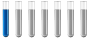total ionic, net ionic equationAuthor Message

NewbieJoined: 18 Mar 2019
Posts: 1# total ionic, net ionic equation

Hello,

I have to make the total ionic and the net ionic equation.

This is my equation: BiI3(s)+KI(aq)=K[BiI4](l)

I started with the total ionic one and this are my results:
BiI3(s)+K^+(aq)+I^-(aq)=

I do not know how I can do this with the complexDistinguished MemberJoined: 27 Sep 2017
Posts: 322
Location: Berlin, GermanyK+(aq) +I-(aq) + BiI3(s) => K+(aq) + [BiI4]-(aq)Display posts from previous: All Posts1 Day7 Days2 Weeks1 Month3 Months6 Months1 Year Oldest FirstNewest FirstPage 1 of 1